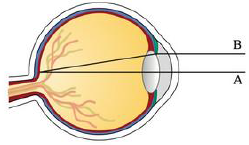Chapter 4.4, Problem 80E### Single Variable Calculus: Early Tr...

8th Edition
James Stewart
ISBN: 9781305270343

#### Solutions

Chapter
Section### Single Variable Calculus: Early Tr...

8th Edition
James Stewart
ISBN: 9781305270343
Textbook Problem

# Light enters the eye through the pupil and strikes the retina, where photoreceptor cells sense light and color. W. Stanley Stiles and B. H. Crawford studied the phenomenon in which measured brightness decreases as light enters farther from the center of the pupil. (See the figure.)A light beam A that enters through the center of the pupil measures brighter than a beam B entering near the edge of the pupil.They detailed their findings of this phenomenon, known as the Stiles–Crawford effect of the first kind, in an important paper published in 1933. In particular, they observed that the amount of luminance sensed was not proportional to the area of the pupil as they expected. The percentage P of the total luminance entering a pupil of radius r mm that is sensed at the retina can be described by P = 1 − 10 − ρ r 2 ρ r 2 ln 10 where ρ is an experimentally determined constant, typically about 0.05.(a) What is the percentage of luminance sensed by a pupil of radius 3 mm? Use ρ = 0.05.(b) Compute the percentage of luminance sensed by a pupil of radius 2 mm. Does it make sense that it is larger than the answer to part (a)?(c) Compute lim x → 0 + P . Is the result what you would expect? Is this result physically possible?Source: Adapted from W. Stiles and B. Crawford, “The Luminous Efficiency of Rays Entering the Eye Pupil at Different Points.” Proceedings of the Royal Society of London, Series B: Biological Sciences 112 (1933): 428–50.

(a)

To determine

To calculate: The value of percentage of luminance sensed by a pupil of radius 3 mm.

Explanation

Given:

The percentage of the total luminance entering a pupil of radius r mm which is sensed by retina is, P=110ρr2ρr2ln10 , where ρ is experimentally determined constant and its value is around 0.05.

Calculation:

Substitute r=3 and ρ=0.05 in P to get the required percentage.

P=110ρr2

(b)

To determine

To calculate: The value of percentage of luminance sensed by a pupil of radius 2 mm and compare the result with the result obtained in part (a).

(c)

To determine

To compute: The value of limit limr0+P and interpret the result.

### Still sussing out bartleby?

Check out a sample textbook solution.

See a sample solution

#### The Solution to Your Study Problems

Bartleby provides explanations to thousands of textbook problems written by our experts, many with advanced degrees!

Get Started